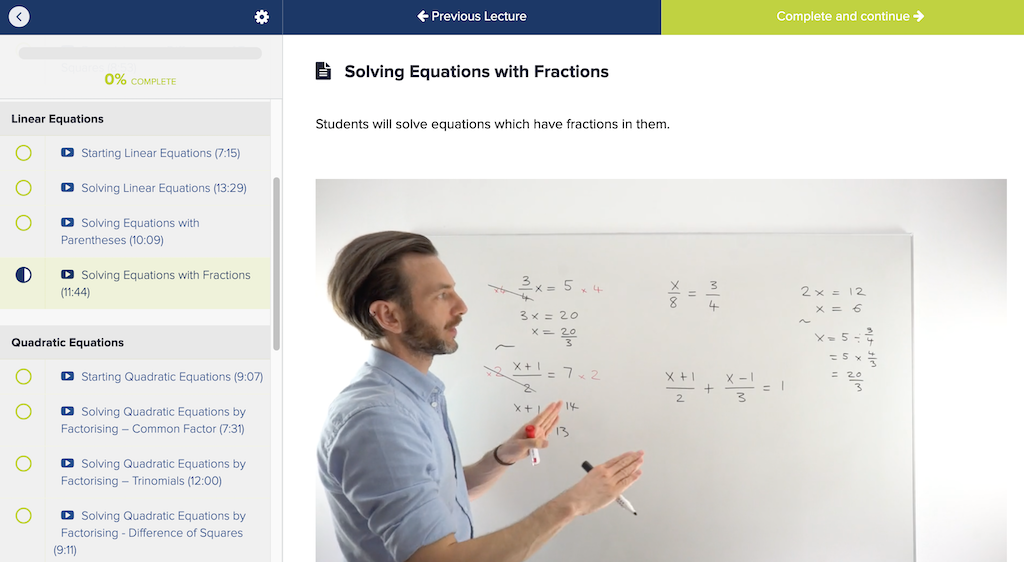# Solving Equations

Equations are everywhere in Math, and there are many different types. Equations are how solutions to Math problems are presented – they’re like the words and sentences of Math! We love seeing students get slick with equations because we know this skill will be with you in all Math classes you ever take. But equations are not easy and each type comes with its own methods of solution, so there’s lots to learn in this online course.

Want to take a sneak peak? Check out a FREE PREVIEW class!We believe in taking students on a learning transformation. So, we’ve bundled this course with others that will be useful in your Algebra to Pre-Calculus journey.

# What You’ll Learn

Starting Equations

Algebraic Expressions

Linear Equations